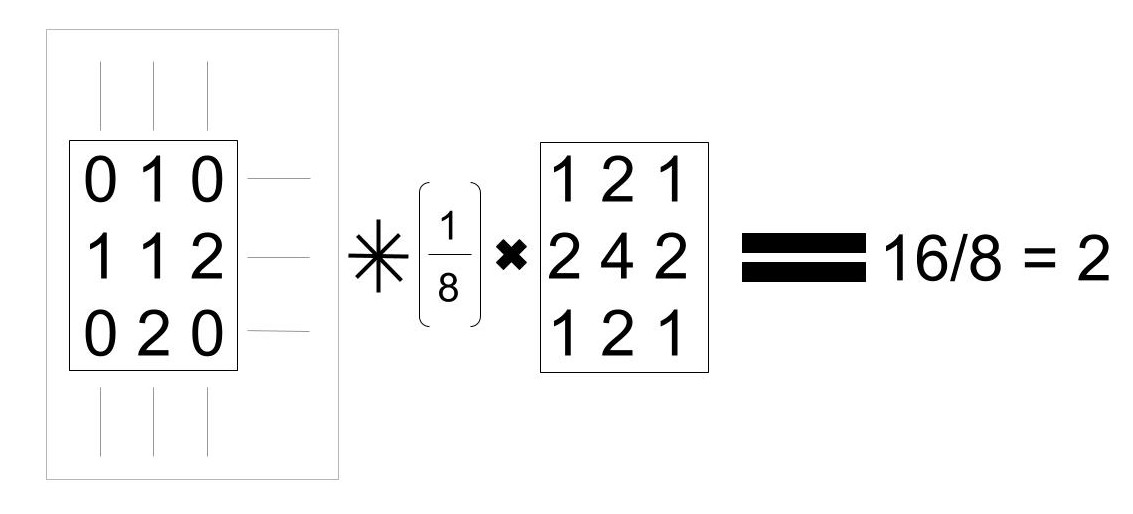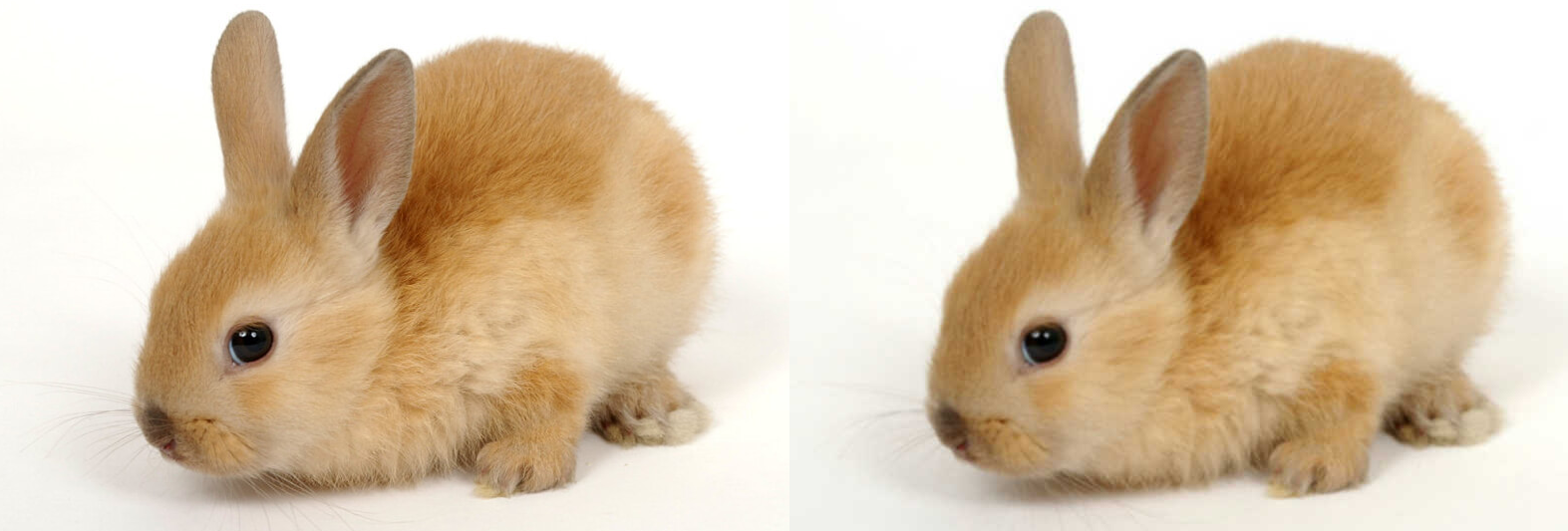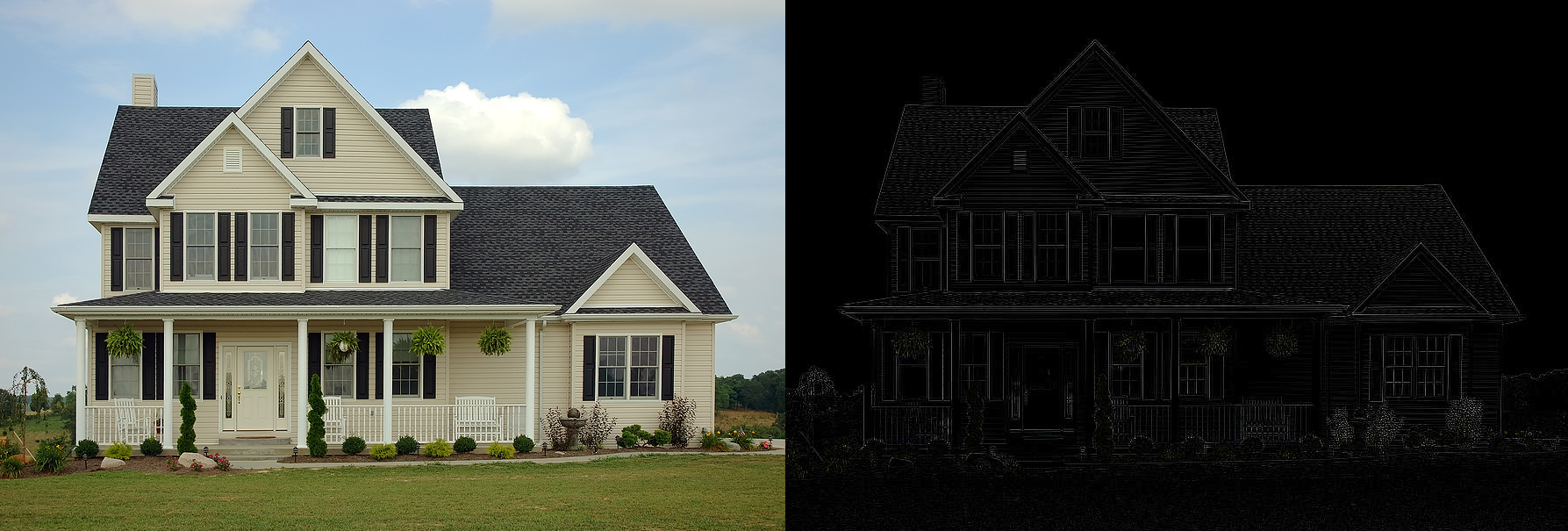# Convolutions in Rust for Deep Learning

29 Apr 2016

In my ongoing attempt to reinvent the wheel I’ve turned my eye to convolutions. Convolutions are a powerful technique in image processing that let you modify images. You have probably seen/used these techniques to blur or sharpen an image. Convolutions also play a key part in deep learning - as I’ll briefly cover in this post.

### What is a convolution?

I’m going to (try to) avoid using too much mathematics in this post. Convolutions are fairly complex but I’ll do my best to keep it simple. There are also some great explanations in my references and elsewhere online.

You can think of a convolution as a filter which passes over an image. We place the filter on a section of the image, put the result in our output image, and then move the filter to the next spot and repeat. These filters are actually matrices representing the convolution. To compute the output we take the element-wise product of the filter with the portion of the image it is covering and then sum the result.

For example say we have the following convolution matrix:

``````       [1 2 1]
1/8  * [2 4 2]
[1 2 1]
``````

This is a `Gaussian Blur` filter. For each block of 3x3 pixels it will produce a new pixel in our output image.A poor diagram explaining convolutions. The left block are the pixels in the image. The right block is our convolution filter. There are clearer diagrams in the articles in references section.

In the above diagram I’m (poorly) depicting the filter acting on a single 3x3 block of pixels in an image. We take a weighted sum of the pixel intensities to produce the output pixel.We have used a gaussian blur convolution on this photo of a rabbit. Nice.

We can get a lot of different effects, for example edge detection:We have used an edge detection convolution on this photo of a house. Also nice.

### How do convolutions tie in to deep learning?

Hopefully the above highlights that convolutions can be very useful for extracting (or dulling) certain features of an image. However, to utilize these filters effectively we often need to do a lot of tweaking.

Enter Neural Networks! Neural Networks are a machine learning algorithm which allows us to learn approximations of unknown functions. The algorithm chains together many layers of weights which when placed together build a network. This network can then be used to infer the unknown function from some data. The idea is that we learn the values of the weights which give the closest approximation to the function. For a slightly less abstract example, let’s imagine that we have lots of photos of cats and dogs. A neural network can be used to automatically identify which photos contain dogs and which contain cats. The unknown function here takes value `0` for a cat photo and `1` for a dog.

So how do convolutions fit in? Instead of setting specific values for our convolution we can choose some unknown weights. These weights can then be learned automatically by the neural network. In this way we completely remove the need to tweak the convolution ourselves. The neural network does all the heavy lifting! The addition of convolutions to neural networks make them a great tool for computer vision. They allow the neural network to automatically learn the relevant features from a set of images.

This is an incredibly powerful and popular technique. Which leads us nicely into the next section.

### Reinventing the wheel

This isn’t a new idea - and you can already find these in Rust! The convolutions exist in Piston’s imageproc . And you can already use Convolutional Neural Networks in autumnai’s leaf .

I’m not doing anything particularly special beyond rewriting all of this stuff completely natively - more fool me.

### What next?

Right now my convolutions work as described above - sliding the convolution matrix over the image. However, in neural networks we want to compute the convolution as a matrix multiplication. This allows us to vectorize the procedure and perform the convolution on many images simultaneously. The next step for me is to efficiently construct the convolution as a matrix multiplication. If people are interested I’d be happy to write about this process in a future post.

Then I have the grueling task of adapting rusty-machine’s current (simple) neural network implementation to support convolutional layers.

This by itself doesn’t quite complete our neural network tool kit. We also need Pooling layers and a few other parts - one step at a time.

##### Piston

I used piston/image to load the images in this demo. I used my own code to handle the convolutions. Thanks Piston devs!